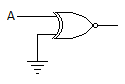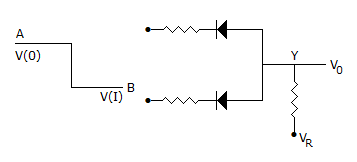# Electronics and Communication Engineering - Digital Electronics

11.

For the gate in the given figure the output will beA. 0 B. 1 C. A D. A

Explanation:

If A = 0, Y = 1 and A = 1, Y = 0 Therefore Y = A.

12.

In the expression A + BC, the total number of minterms will be

 A. 2 B. 3 C. 4 D. 5

Explanation:

The min terms are ABC + ABC + AB C + ABC + ABC.

13.

The circuit in the given figure isA. positive logic XOR gate B. negative logic OR gate C. negative logic AND gate D. positive logic AND gate

Explanation:

Since V(I) is lower state than V(0) it is a negative logic circuit. Since diodes are in reversed parallel, it is an AND gate.

Note: In Boolean algebra it is recognized that a positive logic OR is a negative logic AND. Similarly a positive logic AND is a negative logic OR.

14.

Which of the following is non-saturating?

 A. TTL B. CMOS C. ECL D. Both (a) and (b)

Explanation:

Since it is non-saturating, ECL has low propagation delay.

15.

The number of digits in octal system is

 A. 8 B. 7 C. 9 D. 10